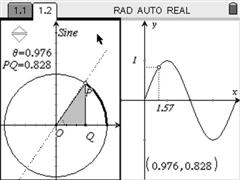PT EN

## Trigonometric CircleSine, Cosine, Tangent and Cotangent of an angle are initially defined as quotients between sides of a triangle. From that definition we go to the trigonometric circle, generalizing the initial definition to angles between 0 and 2Pi and afterwards we study the trigonometric functions.

The goal of this construction is to allow the study the relationship between the definition of the trigonometric quantities in the trigonometric circle and the trigonometric functions, allowing one to observe, for example, the signs of the trigonometric functions in each quadrant.

You can insert this construction in your own documents and therefore create custom activities to your classroom.